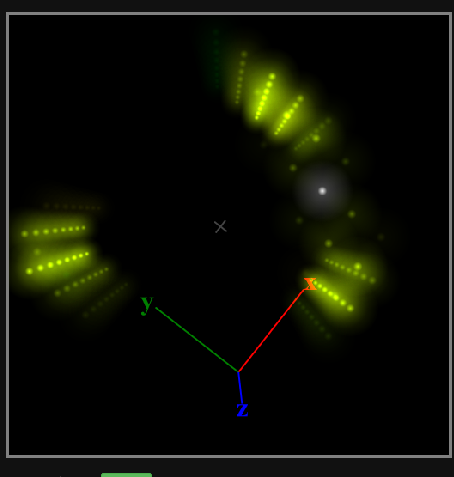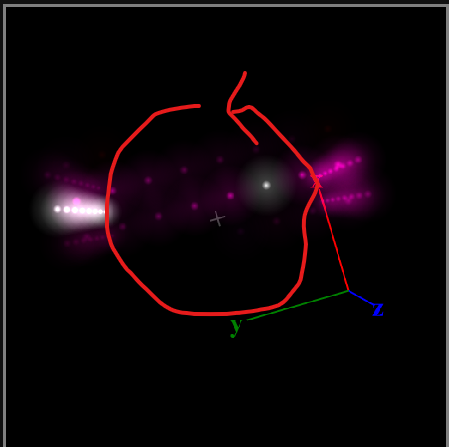# Attempting to constantly rotate a ring from top to bottom of a cube along the Z-axis

Hello! I’m completely new to pixelBlazes, and I’m amazed by how powerful those controllers are!

Expected behavior:

Constantly rotate my weird ring along the z-axis without it dancing around the shape, similar to this gif: Sonic Ring 1991 GIF by augustoodashi on DeviantArt

Rotates for a bit, swaying all over the place, then freezes up. Even the counter stops changing.

I suspect I may be misunderstanding how rotateZ(angs) is supposed to be used. I’ve tried the below code with rotateZ(1) in beforeRender with same results. Is this like an owl turning its head around until its head pops off?

Set-up:

Load a 3D cube into the mapper with ~500 pixels, and run the pattern below. It should look like this:

``````export var rotate
export var pixelCount
export var indexWatcher
export var counter

export function beforeRender(delta) {
/*
This 0..1 time() output cycles every (0.1 * 65.535) seconds. We'll use this
both as the single output hue, as well as a basis for the function that
creates the rotating / bouncing pulse(s).
*/
counter += delta
if(counter > 100){ // 10 times a second
counter = 0
if(rotate > 180)
rotate = 0
else
rotateZ(rotate++)
}
}

export function render3D(index, x, y, z) {
indexWatcher = index
/*
The formula for a 3D plane is:

a(x − x1) + b(y − y1) + c(z − z1) = 0
x y z?

where the plane is normal to the vector (a, b, c). By setting out output
brightness to the right hand side, the initial defined plane is the dark
region, where `v == 0`. This pattern oscillates a, b, and c to rotate the
plane in space. By using the `triangle` function, which is repeatedly
returning 0..1 for input values continuing in either direction away from 0,
we get several resulting 0..1..0.. layers all normal to the vector.

The `3 * wave(t1)` term introduces a periodic phase shift. The final result
is a series of parallel layers, rotating and slicing through 3D space.
*/
v = triangle(x + 0 * y + 0 * z + 0)

// Aggressively thin the plane by making medium-low v very small, for wider
// dark regions
v = pow(v, 15)

// Make the highest brightness values (when v is greater than 0.8) white
// instead of a saturated color
s = v < 0.8

hsv(time(0.1), s, v)
}

``````

Coordinate transformations allow you to manipulate the pixel map coordinates by translating (moving), scaling, and rotating. Up to 31 transformations can be applied. These APIs affect the next render cycle, and can be called in beforeRender or in the main body of code.

You are missiing `resetTransform()`. This resets coordinate transforms to the default. Use this before setting up new transformations.

Without this, you are adding a new transform to the stack every animation frame and will hit the limit quickly.

Also relevant:

rotateZ(`angleRads `)
Rotate 3D space around the Z axis by an angle (in radians).

You’ll probably want to do something like this:

``````resetTransform()
rotateZ(time( 18 / 65.536 ) * PI2) // full rotation every 18 seconds
``````

Ooh, it was indeed the owl’s head getting twisted off! Most excellent, it works now! Thank you.

It still sways around instead of staying in the center. It’s as if the map isn’t centered at 0,0,0. I was expecting the middle point of the line of lit up pixels to stay right at the center where the cross hair is while rotating with the walled cube map.Working rotation pattern below

``````export var rotate
export var pixelCount
export var indexWatcher
export var counter

export function beforeRender(delta) {
/*
This 0..1 time() output cycles every (0.1 * 65.535) seconds. We'll use this
both as the single output hue, as well as a basis for the function that
creates the rotating / bouncing pulse(s).
*/

resetTransform()
rotateZ(time( 18 / 65.536 ) * PI2) // full rotation every 18 seconds
}

export function render3D(index, x, y, z) {
indexWatcher = index
/*
The formula for a 3D plane is:

a(x − x1) + b(y − y1) + c(z − z1) = 0
x y z?

where the plane is normal to the vector (a, b, c). By setting out output
brightness to the right hand side, the initial defined plane is the dark
region, where `v == 0`. This pattern oscillates a, b, and c to rotate the
plane in space. By using the `triangle` function, which is repeatedly
returning 0..1 for input values continuing in either direction away from 0,
we get several resulting 0..1..0.. layers all normal to the vector.

The `3 * wave(t1)` term introduces a periodic phase shift. The final result
is a series of parallel layers, rotating and slicing through 3D space.
*/
v = triangle(x + 0 * y + 0 * z + 0)

// Aggressively thin the plane by making medium-low v very small, for wider
// dark regions
v = pow(v, 15)

// Make the highest brightness values (when v is greater than 0.8) white
// instead of a saturated color
s = v < 0.8

hsv(time(0.1), s, v)
}

``````

(Two posts since I’m still a newbie member, can’t post more than one image in a single post.)Ooh, right, you probably want to move to the center first before rotation!

``````
resetTransform()
translate3D(-.5, -.5, -.5)
rotateZ(time( 18 / 65.536 ) * PI2) // full rotation every 18 seconds
translate3D(.5, .5, .5) //optionally move back so the origin is in a corner instead of the center
``````

Aha, sneaky! Thank you, it works exactly as expected now! I’ll post a video of this running real life LED lights tomorrow night, exciting stuff!

Working Code

``````export var rotate
export var pixelCount
export var indexWatcher
export var counter

export function beforeRender(delta) {
/*
This 0..1 time() output cycles every (0.1 * 65.535) seconds. We'll use this
both as the single output hue, as well as a basis for the function that
creates the rotating / bouncing pulse(s).
*/

resetTransform()
translate3D(-.5, -.5, -.5)
rotateZ(time( 18 / 65.536 ) * PI2) // full rotation every 18 seconds
translate3D(.5, .5, .5) //optionally move back so the origin is in a corner instead of the center

}

export function render3D(index, x, y, z) {
indexWatcher = index
/*
The formula for a 3D plane is:

a(x − x1) + b(y − y1) + c(z − z1) = 0
x y z?

where the plane is normal to the vector (a, b, c). By setting out output
brightness to the right hand side, the initial defined plane is the dark
region, where `v == 0`. This pattern oscillates a, b, and c to rotate the
plane in space. By using the `triangle` function, which is repeatedly
returning 0..1 for input values continuing in either direction away from 0,
we get several resulting 0..1..0.. layers all normal to the vector.

The `3 * wave(t1)` term introduces a periodic phase shift. The final result
is a series of parallel layers, rotating and slicing through 3D space.
*/
v = triangle(x + 0 * y + 0 * z + 0)

// Aggressively thin the plane by making medium-low v very small, for wider
// dark regions
v = pow(v, 15)

// Make the highest brightness values (when v is greater than 0.8) white
// instead of a saturated color
s = v < 0.8

hsv(time(0.1), s, v)
}
``````
2 Likes

Ah, I got back home just a bit ago! 30~ minutes before midnight!

This is what I’ve thrown together using this pattern so far. The sparkle ball is missing its bottom half, making a bigger one soon. I’ve named the prototype sparkle ball `Turtle Catcher`.

That cup with all of the dials in it? `Turtle Catcher Jr`! Throw a pixelblaze(+sensor board extension) into the cup with a power bank, firestorm it up and send data from that pixelblaze to other pixelblazes on the local network to control lights! THE CUP OF POWER!!!

I rigged up the rotation speed of the map to run off the `beats per minute` detection thingy from that crazy music sequencing framework, which seems to be working pretty nicely! Spins when I hit a drum, and spins faster as I drum away faster!

Thank you for the help!

2 Likes